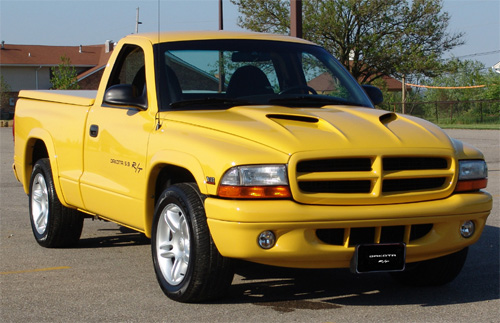# Dodge Dakota R/T Information

###### 1999 Dodge Dakota R/T Information:Above: 1999 Dodge Dakota R/T Solar Yellow Regular Cab. Featured December 2004 on the Mopars Of The Month site. Click Here to check it out.

For 1999 a new color ” Solar Yellow” was added to the list of options for the Dodge Dakota R/T. Production was pumped up with over 6,000 being built. Features remained unchanged from the 1998 model. The Dodge Dakota R/T was a hit, finding one at Dodge Dealers was not easy.

###### 1999 Dodge Dakota R/T Production Numbers

Regular Cab Color:

• Intense Blue: (USA) = 299 – (Canada) = 42 – (Export) = 1 – Total = 342
• Black: (USA) = 679 – (Canada) = 66 – (Export) = 6 – Total = 751
• Deep Amethyst: (USA) = 171 – (Canada) = 12 – (Export) = 0 – Total = 183
• Flame Red: (USA) = 522 – (Canada) = 33 – (Export) = 10 – Total = 565
• Solar Yellow: (USA) = 501 – (Canada) = 76 – (Export) = 10 – Total = 587

Total 1999 Dakota R/T Regular Cab Production = 2,428

Club Cab Color:

• Intense Blue: (USA) = 581 – (Canada) = 132 – (Export) = 2 – Total = 714
• Black: (USA) = 1,394 – (Canada) = 235 – (Export) = 2 – Total = 1,631
• Deep Amethyst: (USA) = 415 – (Canada) = 67 – (Export) = 0 – Total = 482
• Flame Red: (USA) = 1,096 – (Canada) = 127 – (Export) = 6 – Total = 1,229
• Solar Yellow: (USA) = 623 – (Canada) = 160 – (Export) = 5 – Total = 788

Total 1999 Dakota R/T Club Cab Production = 4,844